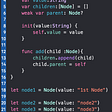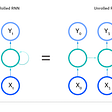# A Stable Numerical Computation

First, we implement the Log-Sum-Exp (LSE) in Python.

`>>> import torch>>> x = torch.randn(3,3)*100>>> x.exp().sum(-1,keepdim=True).log()tensor([[    inf],        [    inf],        [83.7103]])`

In this example, we have tensors that have overflowed while computing LSE.

Now, we use the trick.

`>>> xmax = x.max(-1).unsqueeze(-1)>>> xmax + (x-xmax).exp().sum(-1,keepdim=True).log()tensor([[167.5672],        [165.4862],        [ 83.7103]])`

Finally, we use PyTorch’s implementation of LSE for validation.

`>>> x.logsumexp(-1, keepdim=True)tensor([[167.5672],        [165.4862],        [ 83.7103]])`

As you can see, the outputs are equal.

--

--

--

## More from Mansoor Aldosari

https://github.com/booletic

Love podcasts or audiobooks? Learn on the go with our new app.

## How FLEXable can you be?## ARCUS Common Cache Module with Basic Pattern Caching in Java Environment## Patterns in Usage Equal Happy Users## Tree Data Structure in Swift## Beginners, These Tricks Will Accelerate Your Coding## PPL 2020 — Understanding Agile## Mansoor Aldosari

https://github.com/booletic

## 🧬 Intro to genetic algorithms with python## Time series Forecasting: Using a LSTM Neural Network to predict Bitcoin prices## How to get started with Hyper-parameter Optimization